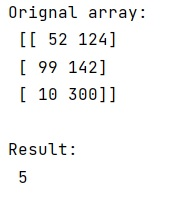# Count all values in a matrix less than a value

Learn, how to count all values in a matrix less than a value in Python?
Submitted by Pranit Sharma, on January 13, 2023

NumPy is an abbreviated form of Numerical Python. It is used for different types of scientific operations in python. Numpy is a vast library in python which is used for almost every kind of scientific or mathematical operation. It is itself an array which is a collection of various methods and functions for processing the arrays.

## Counting all values in a matrix less than a value

Suppose we are given a two-dimensional NumPy array and we need to count all the values that are less than a specific value.

The simple and straight technique to find the count of values is to use as array method with the given array and use the sum() method along with a specific condition. This function converts the input to an array. The input data is in any form that can be converted to an array. This includes lists, lists of tuples, tuples, tuples of tuples, tuples of lists, and ndarrays.

Let us understand with the help of an example,

## Python code to count all values in a matrix less than a value

```# Import numpy
import numpy as np

# Creating numpy array
arr = np.array([[52,124],[99,142],[10,300]])

# Display orinal array
print("Orignal array:\n",arr,"\n")

# asarray
arr = np.asarray(arr)

# Filtering array and finding total values
res = (arr < 150).sum()

# Display result
print("Result:\n",res)
```

Output:What's New (MCQs)

Top Interview Coding Problems/Challenges!

IncludeHelp's Blogs

Languages: » C » C++ » C++ STL » Java » Data Structure » C#.Net » Android » Kotlin » SQL
Web Technologies: » PHP » Python » JavaScript » CSS » Ajax » Node.js » Web programming/HTML
Solved programs: » C » C++ » DS » Java » C#
Aptitude que. & ans.: » C » C++ » Java » DBMS
Interview que. & ans.: » C » Embedded C » Java » SEO » HR
CS Subjects: » CS Basics » O.S. » Networks » DBMS » Embedded Systems » Cloud Computing
» Machine learning » CS Organizations » Linux » DOS
More: » Articles » Puzzles » News/Updates